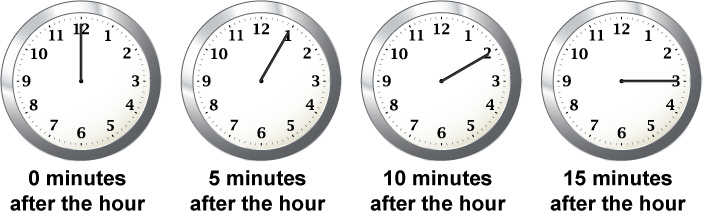# Animated Math Clip Art--Clock Faces--From 10 to 11

This is part of a series of animated math clip art images that show show the time in 15-minute increments. This entire collection covers each of the hours from 12 to 11. Use these animated GIFs with PowerPoint, GoogleSlides, and Media4Math's proprietary Slide Show Creator.

## Telling Time

To review telling time, watch this video. (The transcript is also included.)

Video Transcript

When you look at a clock, you can read the hour and the minutes.

Here is a blank clock face.

Let's start by looking at the hour hand.

It is the shorter of the two clock hands and looks like this.

The hour hand varies in value from 1 to 12.

Here is a tour of times around the clock using the hour hand.

12 o'clock.

1 o'clock.

2 o'clock.

3 o'clock.

4 o'clock.

5 o'clock.

6 o'clock.

7 o'clock.

8 o'clock.

9 o'clock.

10 o'clock.

11 o'clock.

And back to 12 o'clock.

The minute hand is the longer of the two clock hands.

This is what it looks like.

The minute hand varies in value from 0 to 60.

Here is a tour of times around the clock in 5-minute increments.

5 minutes after the hour.

10 minutes after the hour.

15 minutes after the hour.

20 minutes after the hour.

25 minutes after the hour.

30 minutes after the hour.

35 minutes after the hour.

40 minutes after the hour.

45 minutes after the hour.

50 minutes after the hour.

55 minutes after the hour.

0 minutes after the hour.

Telling time involves reading both the hour and minute hand.

Here are some examples.

This is 12 o'clock. Both the hour and minute hands point to 12.

This is 12:15. The hour hand is a bit past 12 and the minute hand points to 3.

This is 12:30. The hour hand is between 12 and 1, and the minute hand points to 6.

This is 12:45. The hour hand is closer to 1 and the minute hand points to 9.

This is 1 o'clock. The hour hand points to 1 and the minute hand points to 12.

This pattern repeats for every hour and minute.

## The Hour Hand

When you look at a clock, you can read the hour and the minutes. Here is a blank clock face.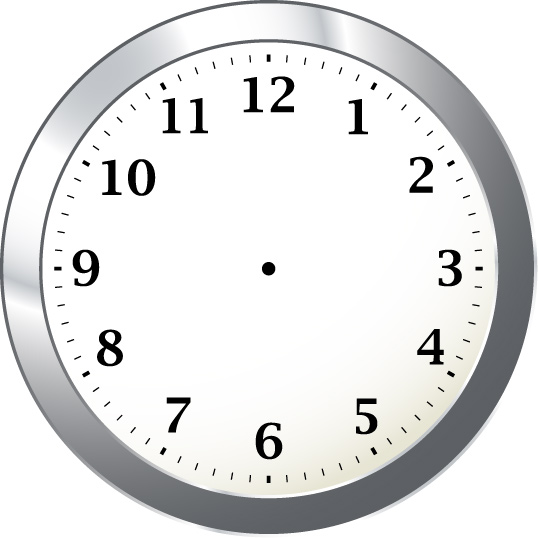Let’s start by looking at the hour hand. It is the shorter of the two clock hands and looks like this.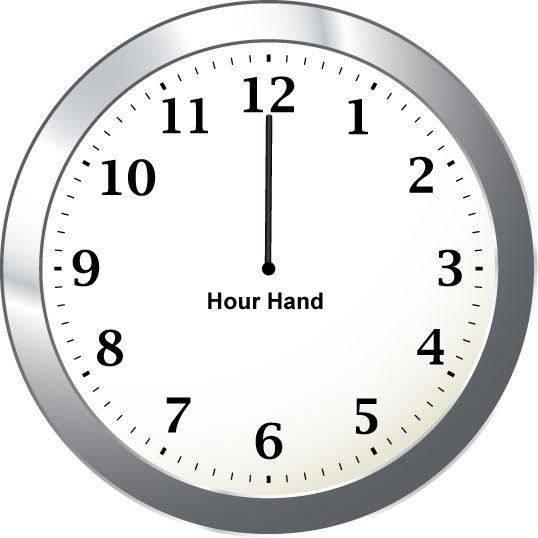The hour hand varies in value from 1 to 12. Here are some sample times using the hour hand.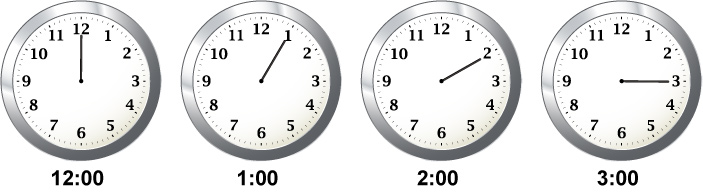## The Minute Hand

The minute hand is the longer of the two clock hands. This is what it looks like.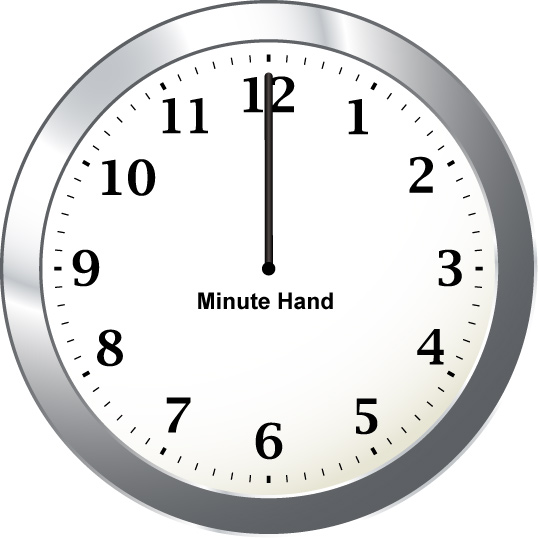The minute hand varies in value from 0 to 60. Here are some sample times using the minute hand.